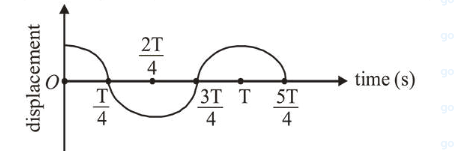# The displacement time graph of a particle executing S.H.M. is given in figure : (sketch is schematic and not to scale)Question:

The displacement time graph of a particle executing S.H.M. is given in figure : (sketch is schematic and not to scale)Which of the following statements is/are true for this motion?

(a) The force is zero at $t=\frac{3 T}{4}$

(b) The acceleration is maximum at $t=T$

(c) The speed is maximum at $t=\frac{T}{4}$

(d) The P.E. is equal to K.E. of the oscillation at $t=\frac{T}{2}$

1. (1) (a), (b) and (d)

2. (2) (b), (c) and (d)

3. (3) (a), (b) and (c)

4. (4) (a) and (d)

Correct Option: , 3

Solution:

(3) From graph equation of SHMv

$X=A \cos \omega t$

(a) At $\frac{3 T}{4}$ particle is at mean position.

$\therefore$ Acceleration $=0$, Force $=0$

(b) At $T$ particle again at extreme position so acceleration ismaximum.

(c) At $t=\frac{T}{4}$, particle is at mean position so velocity is

maximum.

Acceleration $=0$

(d) When $\mathrm{KE}=\mathrm{PE}$

$\Rightarrow \frac{1}{2} k\left(A^{2}-x^{2}\right)=\frac{1}{2} k x^{2}$

Here, $A=$ amplitude of SHM

$x=$ displacement from mean position

$\Rightarrow A^{2}=2 x^{2} \Rightarrow x=\frac{+A}{\sqrt{2}}$

$\Rightarrow \frac{A}{\sqrt{2}}=A \cos \omega t \quad \Rightarrow t=\frac{T}{2}$

$\therefore x=-A$ which is not possible

$\therefore A, B$ and $C$ are correct.